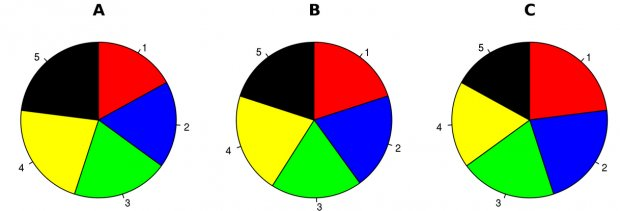# Chapter 2

## Summarizing Data

### Learning Outcomes

• Use appropriate visualizations for different types of data (e.g. histogram, barplot, scatterplot, boxplot, etc.).
• Use different measures of center and spread and be able to describe the robustness of different statistics.
• Describe the shape of distributions vis-a-vis histograms and boxplots.
• Create and intepret contingency and frequency tables (one- and two-way tables).

### Videos

Summarizing and Graphing Numerical Data

Exploring Categorical Data